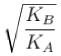Courses

# Simple Harmonic Motion MCQ

## 10 Questions MCQ Test Topic wise Tests for IIT JAM Physics | Simple Harmonic Motion MCQ

Description
This mock test of Simple Harmonic Motion MCQ for IIT JAM helps you for every IIT JAM entrance exam. This contains 10 Multiple Choice Questions for IIT JAM Simple Harmonic Motion MCQ (mcq) to study with solutions a complete question bank. The solved questions answers in this Simple Harmonic Motion MCQ quiz give you a good mix of easy questions and tough questions. IIT JAM students definitely take this Simple Harmonic Motion MCQ exercise for a better result in the exam. You can find other Simple Harmonic Motion MCQ extra questions, long questions & short questions for IIT JAM on EduRev as well by searching above.
QUESTION: 1

### The equation of S.H.M of a particle is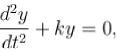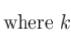is a positive constant. The time period of motion is given by :

Solution:

Compare with equation of SHM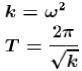The correct answer is: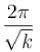QUESTION: 2

### If length of simple pendulum is increased by 6 % then percentage change in time period will be :

Solution: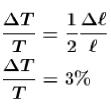The correct answer is: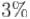QUESTION: 3

### A particle is executing S.H.M. from mean position at 5 cm distance, acceleration is 20 cm/s2 then value of angular velocity will be :

Solution: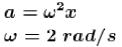QUESTION: 4

A man measures the period of a simple pendulum inside a stationary lift and finds it to be T sec. If the lift accelerates upwards with an acceleration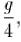then the period of the pendulum will be :

Solution: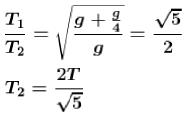The correct answer is: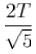QUESTION: 5

The maximum velocity of harmonic oscillator is a, and its maximum acceleration is β Its time period will be :

Solution: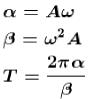The correct answer is: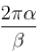QUESTION: 6

One mass m is suspended from a spring. Time period of oscillation is T. Now if spring is divided into n piece and these are joined in parallel order then time period of oscillation if same mass is suspended.

Solution:

Keq = n2k is correct but it is a 2 step process
First, K’ = k x n as the spring constant becomes ‘n’ times due to shortening of length
Second, Keq = n x K’ as they are connected in a parallel manner
=>Keq = n2K

QUESTION: 7

Amplitude of harmonic oscillator is a when velocity of particle is half of maximum velocity, then position of particle will be :

Solution: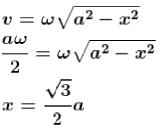The correct answer is: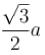QUESTION: 8

A simple pendulum suspended from the ceiling of a stationary trolley has a length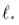Its period of oscillation is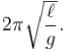What will be its period of oscillation if the trolley moves forward with an acceleration f ?

Solution: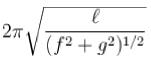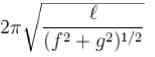QUESTION: 9

In S.H.M, the graph between kinetic energy 'K' and time 't' is:

Solution: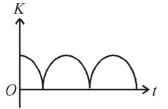QUESTION: 10

Two object A and B of equal mass are suspended from two springs of spring constants KA and KB if the objects oscillate vertically in such a manner that their maximum kinetic energies are equal, then the ratio of their amplitude is :

Solution:

Kinetic energy of A = Kinetic energy of B.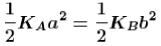So, ratio of amplitude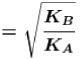The correct answer is: